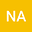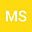ON NEW GENERALIZED NON-INTEGRO-DERIVATIVES AND APPLICATIONS
••• necmettin alp,
• Mehmet Zeki Sarikaya
necmettin alp
Duzce Universitesi
Author ProfileMehmet Zeki Sarikaya
Duzce Universitesi
Author Profile## Abstract

With respect to the non-integro-fractional derivative, in previous studies, the non-integro-fractional derivative of non-negative real numbers can be calculated. However, by previous denitions, the non-integro-fractional de- rivative of negative values can not be calculated due to t; 2 (0; 1). For example, (2)12 =2 R for t = 2 and = 1 2 : So what should we do for the non-integro-fractional derivative of “negative” real numbers? The pur- pose of this paper is to introduce more general derivative denition and we claim that we will obtain non-integro-fractional derivative of “all” real num- bers. Classic derivative, q-derivative, (p; q)-derivative, comformable fractional derivative, Katugampola fractional derivative and backward-forward dierence operator in Time Scale are the special cases of these general derivative deni- tions. These new denitions of ours must give us derivatives on both discrete and continuous calculus.